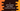# C program to print squares and cubes of all numbers from 1 to n## C program to print squares and cubes of all numbers from 1 to n:

In this post, we will learn how to print square and cube of all numbers from 1 to n in C. Our program will take the value of n as input from the user and print out the list of squares and cubes for each number.

With this program, you will learn how we can use a loop, how we can take user inputs and how to print values in C.

We can solve this problem in different ways. In this post, we will learn how to do that using a for loop and using a while loop.

### C program:

Below is the complete C program:

``````#include <stdio.h>

int main()
{
int i, n;

printf("Enter the value of n : ");
scanf("%d", &n);

printf("Number  Square  Cube\n");

for (i = 1; i < n + 1; i++)
{
printf("%d \t %d \t %d\n", i, i * i, i * i * i);
}

return 0;
}``````

#### Explanation:

In this program,

• i and n are two integer variables. i is used in the for loop and n is used to hold the user input value.
• Using printf, we are asking the user to enter the value of n and using scanf, we are reading that value.
• Using a for loop, we are printing all the numbers, its square value and its cube value from 1 to n. The for loop runs from i = 1 to i = n. Inside the for loop, we are printing the values of i, i * i and i * i * i.

#### Sample output:

This program will give output as like below:

``````Enter the value of n : 9
Number  Square  Cube
1        1       1
2        4       8
3        9       27
4        16      64
5        25      125
6        36      216
7        49      343
8        64      512
9        81      729``````